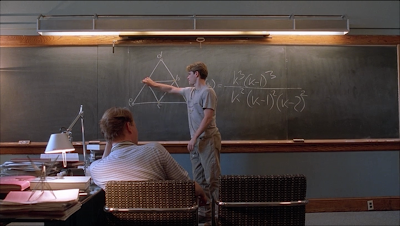Partial Plot Overview:
Will Hunting (Matt Damon) is a 20-year-old living in South Boston and works as a janitor at MIT. However, Will is a genius and displays an ability to absorb information and solve difficult problems with ease. Unfortunately, Will is tried for assault he is put in jail. Fortunately, a math professor at MIT, Gerald Lambeau (Stellan Skarsgård), has recognized Will's talent and has arranged for Will's release provided Will meet with Lambeau as well as see a therapist.Will Hunting (Matt Damon) finishing an eighth (out of ten) homeomorphically irreducible tree with 10 vertices.

After Will has met with and frustrated five different therapists, Lambeau decides to have Will see Lambeau's old college roommate, Sean Maguire (Robin Williams). In any case, the remainder of the movie follow's Will's interactions with his friends, a girl he meets named Skylar (Minnie Driver), Lambeau, and Sean. How will things end for Will? Watch and find out!

[20190218 Edit]

20130310:
This movie is well written. I love the dramatic monologues. Off the top of my head I can remember three: Robin William's story about meeting his wife and the World Series, Ben Affleck's motivational speech to Will, and Robin William's speech to Will in the park. Of course, there were plenty of other great scenes. One of my favorites is the "How do you like them apples?" scene. Another is the "It's not your fault" scene.Will (right) and Chuckie (left, Ben Affleck)

Overall, I highly recommend this movie to anybody who hasn't seen it yet.

Watched several times before.
Watched 20130310 (Netflix, Instant, HD)
Good Will Hunting (1997) Gus Van Sant. 126 min

Good Will Hunting (IMDb.com)
Good Will Hunting (RottenTomatoes.com)
Good Will Hunting (Wikipedia.org)

Mathematics:
Professor Lambeau is teaching a Fourier analysis class. The material on the lecture boards correctly reflects this. However, the problems in the hallway are graph theory problems. No wonder the graduate students in his class can't solve them. :pFirst set of problems.

I tackled some of the problems below, but there's a paper I found that goes into great detail for those who are interested. It's titled Mathematics in Good Will Hunting II: Problems from the Student's Perspective.

First Set of Problems:
1) Find the adjacency matrix $A$.
Answer: The $(i,j)$-th entry of the adjacency matrix gives the number of edges between the $i$th and $j$th vertex. Thus,
$$A = \left[\begin{matrix} 0 & 1 & 0 & 1\\ 1 & 0 & 2 & 1\\ 0 & 2 & 0 & 0\\ 1 & 1 & 0 & 0 \end{matrix}\right]$$
2) Find the matrix giving the number of 3 step walks.
Answer: With an understanding of the information being contained in the adjacency matrix, we can convince ourselves that the number of 3 step walks would be given by $A^3$. In general, the number of $n$ step walks would be given by $A^n$.Skylar (Minnie Driver).

3) Find the generating function for walks from point $i$ to $j$.
Answer: The generating function is the function $f(z)$ such that the coefficient $a_n$ of the expansion $$f(z)=\sum_{i=0}^n a_n z^n$$ gives the number of $n$ step walks from $i$ to $j$. Skipping some explanation, we want to look at $$\sum_{i=0}^n (Az)^n = (1-Az)^{-1}$$ where $A$ is the adjacency matrix from part 1. Thus the generating function for walks from point $i$ to $j$ is given by the $(i,j)$-th entry of $\left(1-Az\right)^{-1}$, which is more explicitly given using Cramer's rule.Sean Maguire (Robin Williams).

4) Find the generating function for walks from 1 to 3.
Answer: By Cramer's rule, we need to compute
$$\frac{(-1)^{1+3}\det\left[\begin{array} -z & 0 & -z\\ 1 & -2z & -z\\ -z & 0 & 1 \end{array}\right]}{\det\left[\begin{array} 1 & -z & 0 & -z\\ -z & 1 & -2z & -z\\ 0 & -2z & 1 & 0\\ -z & -z & 0 & 1 \end{array}\right]}$$
Second Set of Problems:
1) How many trees are there with $n$ labeled vertices?
Answer: This problem is equivalent to counting how many spanning trees for a complete graph with $n$ vertices. Will writes the answer without proof: $$n^{n-2}.$$ See Cayley's formula (Wikipedia.org)Chuckie Sullivan (Ben Affleck).

2) Draw all the homeomorphically irreducible trees with $n=10$.
Answer: A tree is homeomorphically irreducible if it has no vertex of degree two. Let's work our way from $n=1$ up to $n=10$. Let $S_k$ be the star graph with $k-1$ leaves and a root of degree $k-1$.
n=1: $S_1$
n=2: $S_2$
n=3: none
n=4: $S_4$
As we start to think about the problem, we might realize we should focus on the degree of the internal vertices (the vertices which aren't leaves). We can think of each internal vertex as a star and every time we join two of them, then the number of vertices drop by two.
n=5: $S_5$
Now we can start to have more than one internal vertex.
n=6: $S_6$; $S_4$,$S_4$
n=7: $S_7$; $S_4$,$S_5$
Next we can start getting three internal vertices.
n=8: $S_8$, $S_4$,$S_6$; $S_5$,$S_5$; $S_4$,$S_4$,$S_4$
n=9: $S_9$; $S_4$,$S_7$; $S_5$,$S_6$; $S_4$,$S_4$,$S_5$; $S_4$,$S_5$,$S_4$.
Note that in the previous, the order in which I combine the internal vertices matter.
n=10: $S_{10}$; $S_4$,$S_8$; $S_5$,$S_7$; $S_6$,$S_6$; $S_4$,$S_4$,$S_6$; $S_4$,$S_6$,$S_4$; $S_5$,$S_4$,$S_5$; $S_5$,$S_5$,$S_4$; $S_4$,$S_4$,$S_4$,$S_4$ type 1; $S_4$,$S_4$,$S_4$,$S_4$ type 2*
*Type 1 is a chain, while Type 2 has three of the $S_4$'s attached to the fourth $S_4$.Professor Gerald Lambeau (left, Stellan Skarsgard) and Will (right).

The board is missing $S_4$,$S_4$,$S_4$,$S_4$ Type 1 and $S_4$,$S_4$,$S_6$. A reasonable interpretation of the scene is that the Professor scared him away before Will could finish, and that the TA's statement "Looks right" is to mean "Nothing here is wrong" as opposed to "This is a complete answer."

Male Bonding Moment:
I didn't know what they were working on when I watched the movie, but I was able to dig up that Will and Lambeau were computing the chromatic polynomial of the 3-Sun graph. See Sun Graph (mathworld.wolfram.com) and Chromatic Polynomial (mathworld.wolfram.com).